# Kerala Syllabus 9th Standard Physics Solutions Chapter 7 Wave Motion

You can Download Wave Motion Questions and Answers, Summary, Activity, Notes, Kerala Syllabus 9th Standard Physics Solutions Part 2 Chapter 7 help you to revise complete Syllabus and score more marks in your examinations.

## Kerala State Syllabus 9th Standard Physics Solutions Chapter 7 Wave Motion

### Wave Motion Textual Questions and Answers

Wave Motion

Fill half of a trough with water. Place some crumpled paper balls in it. Make ripples on the surface of the water using finger.
Observation:
We can see disturbance spreading from its origin to other place. Water particles move up and down about their mean position without displacement in the direction of propagation of wave. Energy is transferred from particle to particle and spreads everywhere due to wave motion. Wave motion is the propagation of disturbances, produced on one part of a medium by the vibration of its particles, to all its other parts.

Question 1.
Write down examples of wave motion that you see around.

• waves on water
• waves formed in a slinky
• waves formed when a rope is moved up and down after tying one of its ends.
• waves formed on stretched strings.Mechanical waves & electromagnetic waves:
Waves can be classified mainly into two types

1. Mechanical waves: The presence of a medium is required for the transmission of these waves, eg. The waves formed on the surface of water, sound waves etc.
2. Electromagnetic waves: Electromagnetic wave is a combined form of an electric field and a magnetic field which vary continuously. A medium is not essential for its propagation

Question 2.
How many types of mechanical waves are there? What are they?
There are two types of mechanical waves:
They are

1. Transverse waves
2. Longitudinal waves

Transverse Wave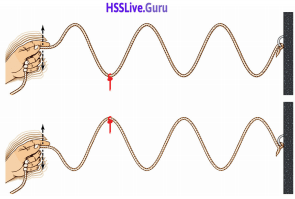Tie one end of a rope to a window, Wind a ribbon or paper on the rope ins such a way that you can see it clearly. Hold the other end of the rope and move it up and down. Observe the wave motion on the rope.

Question 3.
How does the ribbon/paper move?
Ribbon/ paper moves up and down

Question 4.
In which direction does the wave move?
The wave moves forward or horizontally.

Inferences

1. The ribbon moves up and down
2. The ribbon’s position on the rope does not change.
3. The ribbon is vibrating in a direction perpendicular to the direction of propagation of the wave.
Each particle of the wave vibrates in a direction perpendicular to the direction of propagation of the wave.4. In this case is the motion of particles parallel or perpendicular to the direction of propagation of the wave? The direction of motion of particles is perpendicular to the direction of propagation of the wave. A transverse wave is a wave in which the particles of the medium vibrate in a direction perpendicular to the direction of propagation of the wave.

Can’t you explain why the disturbances on water could not make the paper boat move away from the shore? The waves formed on the surface of water is transverse wave. The water particles move only up and down. The water particles do not move in the horizontal direction. So the paper boats could not move away from the shore.

Observe the graphic representation of a transverse wave at a particular instant.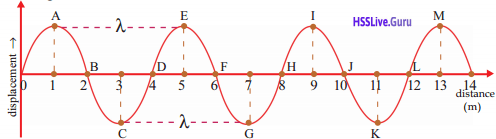Question 5.
What are crests and troughs?
The elevated portions are called crests. The depressed portions are called troughs

Question 6.
In the figure, which are the points of the highest displacement (amplitude)?
A, C, E, G, I, K, M

Question 7.
How many crests and troughs are there in the figure?
4 crests, and 3 troughs.

Question 8.
Whether all the particles are in the same phase of vibration at a particular time?
No

Question 9.
Which are the particles in the same phase of vibration as that of A?
E, I, M

Question 10.
G, K

Question 11.
What is the wavelength of the wave shown in the figure?
Wavelength = 4 m

Characteristics of Waves:

1. Amplitude : Amplitude is the maximum displacement of a particle from its mean position. This is denoted by the letter a.
2. Wavelength: Wavelength is the distance between two consecutive particles which are in the same phase of vibration. This is equivalent to the distance advanced by the wave by the time a particle has completed one vibration. The Greek letter X (lambda) is used to denote the wavelength. The unit is metre (m).
3. Frequency: Frequency is the number of vibrations in one second.
Frequency = $$\frac{\text { number of vibrations }}{\text { Time taken }}$$$$\mathrm{f}=\frac{\mathrm{n}}{\mathrm{t}}$$
The unit of frequency is hertz (Hz).

Question 12.
What is the frequency of the wave if the particles A makes 100 vibrations is 5 s?
$$\begin{array}{l}{\text { Frequency of the wave }=\frac{\text { number of vibrations }}{\text { time }}} \\ {f=n / t=\frac{100}{5}=20 \mathrm{Hz}}\end{array}$$
The equation connecting velocity, wavelength, and frequency of a wave is v=f λ ;
v – Velocity (distance travelled by the wave in one second);
f – frequency (number of vibrations in one second);
x – wavelength (distance between two consecutive particles which are in the same phase of vibration).

The graphical representation of two waves of the same amplitude, generated at specific intervals of time, is given below.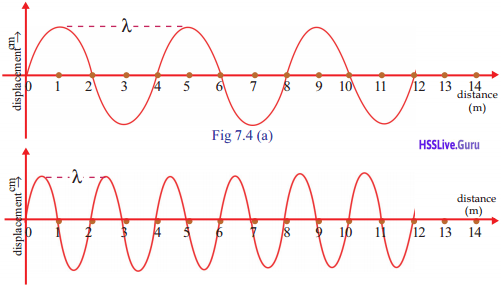Question 13.
What is the wavelength of the first wave? What about the second one?

• wavelength of the first wave = 4m
• wavelength of the second one = 2m

Question 14.
Which wave has a higher wavelength?
First wave has a higher wavelength.

Question 15.
Calculate the frequency of each wave if they have traveled this distance (12 m) in 0.25s.
Frequency of the first wave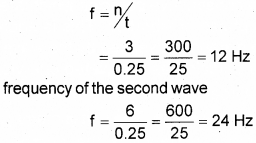Question 16.
What change takes place in the wavelength when the frequency increases?
As frequency increases, wavelength decreases. Wavelength of a wave with a constant speed decreases with increase in frequency, ie. frequency is inversely proportional to the wavelength.
f ∝ 1/λQuestion 17.
Observe the graphic representation of a wave motion given below.a) What is the amplitude of the wave?
b) What is the wavelength?
c) Calculate the frequency of the wave if it took 0.2s to reach A.
d) Calculate the speed of the wave
a) 2 cm
b) 8 m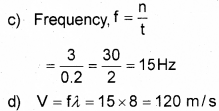Longitudinal Wave

Fix one end of a slinky to a wall. Hang some pieces of paper on the coils at equal distances. Press a few coils on the free end held in the hand and then release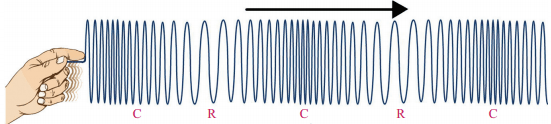Observation: The air particles vibrate to and fro when the waves pass through air.
High pressure is experienced in places where the air particles are close. Such a region is the compression (C). Regions of low pressure are the rarefactions (R).

A longitudinal wave is a wave in which the particles of the medium vibrate in a direction parallel to the direction of propagation of the wave. This creates compressions and rarefactions alternately in the medium.

Question 18.
How do we hear a sound from a source?
Listen to the sound from an excited tuning fork. The vibrations of the tuning fork make the air particles around it to vibrate.
Sound waves are longitudinal wavesQuestion 19.
How many compressions are their in the longitudinal wave shown in the figure?
4 compressions

Question 20.
Find out the differences between transverse and longitudinal waves and complete the table.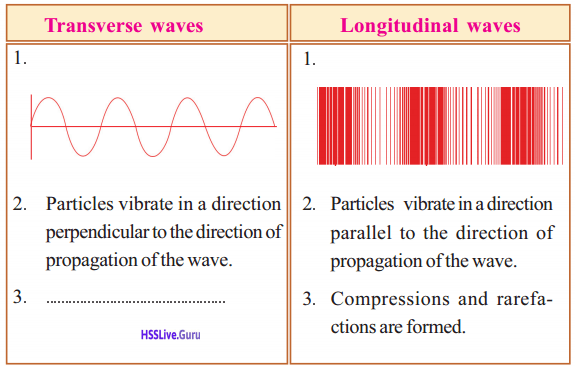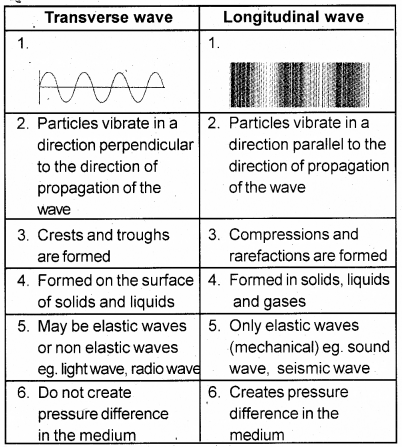Sound

Sound is produced by the vibration of objects. Sound is a longitudinal wave. Sound needs a material medium to travel.Question 21.
What do C and R in the figure indicate?
C for compressions and R for rarefactions.
Wavelength of longitudinal wave The distance between corresponding points of two consecutive compressions or two consecutive rarefactions is the wavelength of the longitudinal wave.

Question 22.
Find out the wavelength in the figure and write it down.
Wavelength = 1 m

Question 23.
What is the speed of the wave if its frequency is 92 Hz?
Velocity V = f λ = 92 × 1 = 92 m/s

Speed Of Sound

• Sound travels through all media.
• The speed of sound differs from one medium to another.
• Sound waves involve the vibrations of the particle of the medium. So sound waves can not travel through vacuum.
• Sound travels faster in solids because the mol¬ecules are closely packed.
• Speed of sound in liquids is comparatively slow.
• Speed of sound in gases is much slower because molecules are loosely packed.
• One of the reasons for the difference in the speed of sound is the difference in the density of the media.
 Medium Velocity(m/s) (At 20°C) Solid Aluminum 6420 Steel 5941 Liquid Pure water 1482 Seawater 1522 Gas Air 343 Helium 965

Question 24.
What are the factors that influence the speed of sound through air?

• Humidity [As humidity increases, speed increases)
• Density [As density increases, speed decreases]
• Wind
• Temperature [As temperature increases, speed increases] ‘
• Nature of the medium.

Humidity and speed of sound:
The amount of water vapor in the air is humidity. It is less during winter and high in summer. The speed of sound increases with increase in humidity. This is because the density of air decreases with the increase in humidity.

Reflection Of SoundArrange two PVC pipes, a glass plate, and a stop clock as shown in the figure.
We can hear sound through the pipe B.
It is due to the reflection of sound from the glass plate.
Sound can also reflect like light The law of reflection, ∠i = ∠r is true in the case of sound also.

Multiple Reflection Of Sound

Sound getting reflected repeatedly from different objects is multiple reflection.

Situation making use of multiple reflection:

• Devices like megaphone, horns, musical instruments like shehnai and trumpets, are made in such a way that the sound produced form them travels only in a certain direction without spreading to other directions. In such devices there is a conical shaped open end which enables the reflected sounds to travel in a particular direction alone, thus enabling us to hear it louder.
• Stethoscope: Helps us to detect beats in the body especially heartbeats.
• The ceilings of halls are given a curvature: As a result sound undergoes multiple reflection and spreads everywhere in the hall.
• Soundboards: The curved soundboards placed behind the screen makes the sound undergo multiple reflections and spreads everywhere in the hall. The boards in musical instruments like guitar, violin, etc., also act as soundboards.

Reverberation

Reverberation is the persistence of sound as a result of multiple reflection

Question 25.
If we felt a boom of sound in empty rooms.
a) Which are the regions where sound waves in a room get reflected?
b) Do these repeatedly reflected sound waves reach the ear of a listener simultaneously?
c) Will you be able to hear all these sounds clearly due to the persistence of audibility? Won’t’ you be hearing only a boom of all the sound?
a) The sound waves get reflected form the walls, ceilings, floor etc.
b) No
c) We will not be able to hear all these sounds clearly, due to the persistence of audibility. We will be able to hear only a boom of all the sounds. This is as a result of reverberation.Persistence of Audibility:
The sensation of hearing produced by a sound is 1
retained for a period of 1/10 s = 0.1 s. This characteristics of the ear is the persistence of audibility. If another sound reaches the ear within 0.1 s, simultaneous hearing of both the sounds is experienced.

Echo

The phenomenon of hearing a sound by reflection from a surface or obstacle, after hearing the original sound is the echo.

Question 26.
What should be the minimum distance to the reflecting surface if the velocity of sound in air is 340 m/s?
Due to persistence of audibility, we can hear the first sound and reflected sound separately, only if there is a time gap second between them.
Speed = $$\frac { Distance travelled }{ time }$$
Speed of sound in air = 340 m/s
time = 1/10 s
Distance = speed × time
= 340 × 1/10
= 34m
So the minimum distance to the reflecting surface is half of 34 m.
i.e. = 17 mQuestion 27.
A person who bursts a cracker hears its echo after 1 s. What is the distance to the reflecting surface if the speed of sound in air is 340 m/s?
2d = v × t = 340 × 1 = 340
∴ d = 340/2 = 170m

Question 28.
What should be the minimum distance between the source and the reflecting surface in water to identify the echo within water? (speed of sound in water is 1482 m/s)
Velocity sound = 1482 m/s
Distance = velocity × time
= 1482 × 1/10
= 148.2 m
So the minimum distance between the source and the reflecting surface in water to identify the echo within water
= 148.2/2
= 74.1 m

Question 29.
Write down the situation in which echo is heard.
When we clap our hands from a field or valley having width greater the 17m, we can hear echo. When we talk loudly standing in an auditorium having length more than 17m, we can hear echo.

Acoustics Of Buildings

Acoustics of building is the branch of science that deals with the conditions to be fulfilled in the construction of a building for clear audibility.

Question 30.
Why are the walls made rough in big halls like the cinema theatres?
The walls are made rough to avoid the regular reflection of sound. Rough surfaces can absorb sound.

Question 31.
With respect to reflection of sound, what are the problems if the distance between the walls in a room is more than 17 m?
If the distance between the walls in a room is more than 17m, the sound waves are reflected repeatedly from the walls, ceiling and floor of the hall, and produce many echos So the sound becomes blurred, distorted and confusing due to overlapping of different sounds.

Question 32.
What are the methods to minimize the problems that occur due to reflection of sound?
The methods to minimize the problems that occur due to reflection of sound are

• Use curtains having many folds.
• Provide large number of ventilators and windows.
• Make the walls and roof rough.
• Make sure that the ratio between the height and width of the hall 2:3.
• Make the floor rough using carpets.
• Increase the number of audiences.

Whispering galley:
The Whispering Gallery at St. Paul’s Cathedral in London is the best example for the reflection of, sound. Event if you are only whispering near the circular wall below the dome the sound will be heard loudly anywhere within the gallery. This is due to the multiple reflection of sound from the circular walls. The Gol Gumbas in Bijapur of Karnataka is another example.

Ultrasonic Sound

Sound with a frequency greater than 20000 Hz, ie, above the higher limit of audibility is called ultrasonic sound.

Uses of Ultrasonic waves:
1. Ultrasonic waves are used to clean spiral tubes, machine parts without a definite shape and electronic components. Objects to be cleaned are dipped in a cleaning solution. Ultrasonic waves are passed through this solution. Due to the high frequency of vibration of ultrasonic waves, dust and grease like substances get detached and are removed from the object.2. Ultrasonic waves are used to detect cracks and flaws in large metal blocksUltrasonic waves passed through a metal block, are allowed to reach the detectors. If there is any crack or flaw, ultrasonic waves will reflect back from that part. So they do not reach the detectors. Audible sound waves of longer wavelength cannot be used for this purpose as they bend around the corners of the defective location to reach the detectors.
3. Echocardiography: Ultrasonic waves are used for taking images of heart. This is known as Echocardiography (ECG).
4. Ultrasonography: Ultrasonic waves are used for getting images of internal organs such as kidney, liver, gall bladder and uterus. Ultrasonic waves travel through the tissues of the body and get reflected from the region where there is a change in tissue density. These waves are then converted into electrical signals and are used to form images of the organs. This technology is called ultrasonography Ultrasonic waves can crush small stones formed in the kidney into fine grains.
5. Sonar (Sound Navigation and Ranging): Sonar is a device that uses ultrasonic waves to measure the distance, direction, and speed of objects underwater.In the figure, ultrasonic waves are sent out from a SONAR which is installed in a ship and they get reflected back after striking an object at the bottom of the sea.

Question 33.
What happens to the ultrasonic waves after striking the object on the seabed?
Ultrasonic waves that are reflected back after striking the object reach the detector. The detector converts the ultrasonic waves into electrical signals.

Question 34.
The distance traveled by a wave can be calculated by knowing the speed of ultrasonic sound in seawater and the time taken for the wave to return.
Distance = speed × timeQuestion 35.
Ultrasonic waves from a ship hits a rock at the bottom of the sea and comes back after 0.5 s. Calculate the distance to the rock from the ship. Consider speed of sound through seawater as 1522 m/s.
Distance = speed × time .
= 1522 × $$\frac { 0.5 }{ 2 }$$ = 380.5 m

Question 36.
Bats make use of ultrasonic sounds for catching prey. How do bats catch prey? Observe figure and write down in your science diary.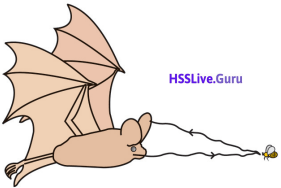Bats produce ultrasonic sounds and the sound gets reflected after striking the prey. It can receive the waves.

Seismic Waves And Tsunami

Originate from the epicenter of the earthquake. Seismology is the branch of science that deals with the study of seismic waves. Scientists dealing with the study of seismic waves are called seismologists. The intensity of earthquake is measured in Richter scale.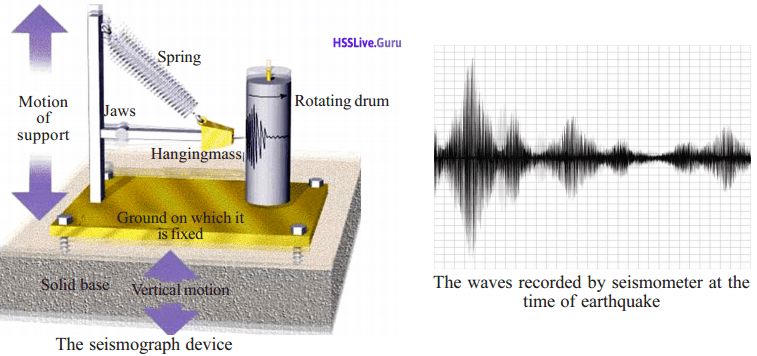Seismic waves formed as a result of earthquakes are classified into three: Primary waves (p waves), Secondary waves (S waves) and surface waves. Among these, primary waves travel fastest.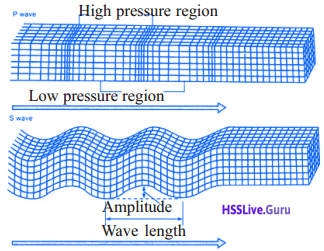Secondary waves are slower than the primary waves. In a seismograph, the difference in the arrival time of the primary and secondary waves can be used to determine the approximate distance to the epicenter. The amplitude of the waves, obtained using seismo-graph determines the intensity of an earthquake. Two surface waves, Rayleigh waves that travel only through the Earth’s surface and Love waves, are also formed as a result of earthquake. Surface waves are the reason for major damages caused by earthquake, though the speed-of surface waves is less than that of secondary waves.

Tsunami

Tsunami is a series of gigantic waves in a water body caused by the displacement of large volume of water in the deep regions near the sea bed. Tsunamis are caused by underwater earthquakes, volcanic eruptions, meteorite impacts, and other such disturbances. The term

Tsunami is coined by combining two Japanese words Tsu’ which means ‘harbor’ and ‘nami’ .which means ‘long wave’. In the bay region, the speed of Tsunami ranges from 600 to 800 km/h and their wavelength from 10 to 1000 km. The amplitude is less in the deep sea. Hence Tsunami is not felt by passengers . in ships. As waves approach the seashore, the trough of the waves rubs against the land. As a result the speed and wavelength of the waves drop down suddenly, amplitude increases and the coastal re¬gion gets submerged.

Tsunami height depends on the geographical nature of the coast and the depth of the seabed. As Tsu¬nami approaches the shore from the deep sea, the energy lost is not significant. Hence the magnitude of destruction will be very high. If it is the crest of the wave that first reaches the shore, the waves rise high and if it is the trough that reaches first, the sea will be in a state of retreat. The system that gives advance warning about Tsunami is known as DART (Deep Ocean Assessment and Reporting of Tsunami).

Question 37.
What are the methods to be adopted to escape from Tsunami? Discuss.

• Move to a higher plain taking the unusual receding of the sea from the sea shore as a warning of the approaching Tsunami.
• Don’t assume for yourself that the danger is over, instead, wait for the official announcement.
• Try to save your life and not your belongings, as life is precious.
• If caught in Tsunami, try to save yourself by latching onto some floating objects.

Let Us Assess

Question 1.
Observe the graph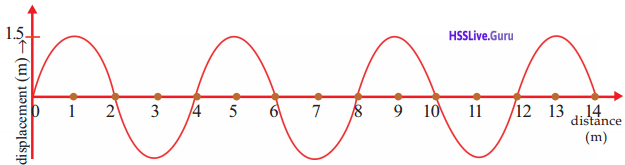1. Find out the amplitude of the wave
2. What is the speed of the wave if it travels 800 m in 2 s?
3. What is the frequency of the wave?
1. The amplitude = 1. 5 m
2. Speed =  $$\frac { Distance travellaed }{ time }$$
3. V = 800/2 = 400 m/s
λ = 4m
v= 400 m/s
f = V/ λ = 400/4 = 100 Hz

Question 2.
What do you mean by acoustics of buildings? Suggest four steps that can be taken, while constructing buildings, to avoid problems that may occur due to multiple reflection of sound.
Acoustics of buildings is the branch of science that deals with the conditions to be fulfilled in the construction of a building for clear audibility.
To avoid problems that may occur due to multiple reflection of sound are

• Make the floor rough using carpets.
• Provide large number of ventilators and windows
• Make the walls and roof rough.
• Use curtains having many folds.

Question 3.
A sound signal from a ship floating on water hits a rock at the bottom of the sea and comes back to the ship after 4s. Calculate the distance of the rock from the surface of water. The speed of sound in water is estimated to be 1500 m/s
V = s/t
Velocity of sound = V = 1500 m / s
time ,t = 4s
Distance travelled, S = v x t =1500 x 4 = 6000 m
= 6000 m
Distance of the rock from the surface of water = 6000/2 m
= 3000 m

Question 4.
Wavelength of a wave that travels with a speed 339 ms is 1.5 km. What will be its frequency?
v = 339 m/s
λ = 1.5 km
= 1500 m
V = f λ
f = v/λ = 339/1500
= 0.226 Hz

Question 5.
Wavelength of a sound wave having frequency 2 kHz is 35 cm. How much time will it take to travel a distance 1500 m?
f = 2 kHz = 2000 Hz
λ = 35 cm = 0.35 m
v = f λ = 2000 × 0.35
= 700 m/s
t = $$\frac{\text { distance }}{\text { time }}$$
= $$\frac { 1500 }{ 700 }$$ = 2.14 s

Question 6.
For a person with normal hearing, the limit of audibility is 20 Hz to 20000 Hz. If so, what will be the limit of wavelength of sound waves that are audible to human beings? Assume that the speed of sound is 340 m/s.
f = 20 Hz,
v = f λ ,
λ = v/f = 17m
f = 20000Hz,
v = f λ,
λ = 0.017 m
so limit of wavelength = 0.017 to 17
= 0.017 to 17 m### Wave Motion More Questions and Answers

Question 1.
Classify the following statements as transverse waves and longitudinal waves.
a) Particles of the medium vibrate perpendicular to the direction of propagation of the wave.
b) Creates pressure difference in the medium
c) Forms in solids, liquids, and gases
d) Lightwaves
e) Seismic waves
f) Forms crest and trough
Transverse wave: a, d, f
Longitudinal wave: b, c, e

Question 2.
Observe the figurea) What kind of wave motion is shown in the figure? Illustrate your answer
b) Calculate the frequency of this wave if its velocity is 6420 m/s and wavelength is 6 m.
a) Longitudinal wave
Here pressure difference is plotted against the y-axis. Pressure difference forms only in longitudinal waves
b) V = 6420 m/s
λ = 6 m
frequency, f = V/λ
= 6420/6 = 1070 Hz

Question 3.
If a person clap his hands stands at a distance of 99m from a wall hear the echo after 0.6s, what is the velocity of sound?
Distance travelled by the sound
= 2 x 99 = 198 m
time = 0.65
Velocity = $$\frac{\text { Distance }}{\text { time }}$$
V = 198/0.6
= 330 m/s

Question 4.
A sound signal of 50 kHz is sent to the bottom of a sea. It returned back after 4S. The velocity of sound in seawater is 1500 m/s. Then,
a) Calculate the depth of the sea
b) What is the wavelength of the wave?
a) Suppose the depth of the sea =d
then distance travelled by the wave = 2d
distance – velocity × time
= 1500 x 4 = 6000m
∴ depth = 6000/2
= 3000 m
b) V = f λ
V = 1500 m/s
f = 50 KHz
= 50000Hz
λ = $$\frac{v}{f}=\frac{1500}{50000}=0.03 \mathrm{m}$$

Question 5.
Given below are graphs of sound waves from differ¬ent sources that travels through the same medium. Among these, which one has higher frequency? What is the basis of your conclusion?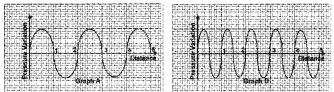Wave with higher frequency is shown in graph B.
As both travel through the same medium, velocity remains the same. But wavelength is inversely proportional to the frequency. ‘B’ has lower wavelength, so B itself has higher frequency.

Question 6.
A sound wave enters water from air. What happens to its wavelength? Why?
Wavelength increases. Velocity of sound in water is 1482 m/s and velocity in air is 343 m/s. But the frequency of the sound wave will not change as the medium differs. We know V = f λ. So wavelength should increase.

Question 7.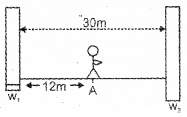A person standing at A claps his hands,
a) Is there any change for occurring echo?
a) Yes, can hear echo.Question 8.
Figure shows the distance – displacement graph. The wave is formed in 2s.a) What is its amplitude?
b) What is its wavelength?
c) What is its frequency?
d) What is its velocity?
a) 0.2 m
b) 4 m
c) f = $$\frac { n }{ t }$$ = $$\frac { 4 }{ 2 }$$ = 2Hz
d) V = f λ = 2 × 4 = 8 m/s

Question 9.A: Stethoscope
B: Reverberation C: Echo
D: 1) Provide more ventilation and windows
2) Use carpets on the floor

Question 10.
Figure shows the distance and displacement of a wave formed in 0.2s.a) What is its wavelength?
b) What is the frequency?
c) What is its velocity?
a) 5 m
b) f = $$\frac { n }{ t }$$ = $$\frac { 3 }{ 0.2 }$$ = 15Hz
c) V = f λ = 15 × 5 = 75 m/s

Question 11.
Identify what kind of wave the following are
a) sound wave
b) ripples formed on the surface of water.
c) wave formed due to vibration of tuning fork.
a) longitudinal wave
b) transverse wave
c) longitudinal wave

Question 12.
Observe the figurea) Which particle is in the same phase of vibration as that of B?
b) What is the distance between these particles called?
c) If the distance between C and E is 25 m, what is the wavelength?
a) F
b) wavelength
c) 50 m

Question 13.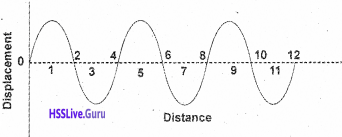a) How many trough are there?
b) Find out the wavelength?
c) Calculate velocity of the wave if it is traveled within 0.02 S.
a) 2
b) 4 m
c) f = $$\frac { n }{ t }$$ = $$\frac { 3 }{ 0.02 }$$ = $$\frac { 300 }{ 2 }$$ = 150 Hz
V = f λ
= 150 × 4 = 600 m/s

Question 14.a) What is its amplitude?
b) What is the frequency?
c) Draw the graph of another wave with no change in the frequency and with half of its amplitude.
d) If this wave travels with velocity 300m/s in 1s, calculate the wavelength?
a) 0.1 m
b) f = $$\frac { n }{ t }$$ = $$\frac { 2 }{ 4 }$$ = 0.5 Hz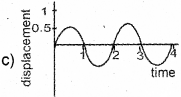d) V = 300 m/s
V = f λ
f = 0.5 Hz
= 300 = 0.5 × λ
λ = 300/0.5 = 600 m

Question 15.
If a sound wave travels 1700 m through a medium in 5 s. Identify the medium. (March 2016)
Air
V = $$\frac { s }{ t }$$
= $$\frac { 1700 }{ 5 }$$ = 340 m/s

Question 16.Given in the graph the points A, B, C, D represents state of vibration of a sound wave. From the below-mentioned options which represent the wavelength.
a) Distance between A and C
b) Distance between A and D
c) Distance between A and B
d) Distance between B and C
Distance between A and CQuestion 17.
Select the instrument that works on the principle of multiple reflection of sound,
a) Watthour meter
b) Sonar
c) Stethoscope
d) Decibel meter (March 2015)
c) Stethoscope

Question 18.
State what happens to the loudness of sound in the following cases.
a) Density of the medium increases.
b) Distance between the source and the receiver increases.
a) Loudness increases (March 2015)
b) Loudness decreases

Question 19.
A sound wave generated in 4 seconds is shown in the graph.If wavelength of the wave is 15m, calculate
a) Frequency of the wave
b) Distance traveled by the wave in 4 seconds.
c) Find out the amplitude of the wave. (March 2015)
a) 1 Hz.
b) 60m
c) 1m

Question 20.
A sound was produced. Three seconds later, its echo was heard.
a) What is the distance between the reflecting surface and source? (Speed of sound in air is 340 m/s)
b) Write TWO methods, by which you can reduce the harmful effects of reflection of sound in big halls. (March 2015)
a) Distance = Velocity × time
= 340 × 3 = 1020m
So distance between the source and reflecting surface = 1020/2 = 510 m
b) Make the walls rough, provide a large number of ventilators. .

Question 21.
State what change happens to the loudness of sound in the following situations:
a) Amplitude of vibration decreases.
b) The distance between source and receiver decreases (Model 2014)
a) Loudness decreases
b) Loudness increases

Question 22.
The wavelength of sound traveling in air with the velocity 330 m/s was found to be 66 m. If so.
a) Find the frequency of sound.
b) By what name are sounds of such frequency known as? (Model 2014)
a) V = f λ
330 = f × 66
f = 330/60 = 5.5 Hz
b) Infrasonic ( ∠ 20Hz)

Question 23.
Velocity of sound in air is 340 m/s. Sound waves of wavelength 0.01 m. from a vibrating body reach your ear through air. Will you be able to hear the sound? Justify your answer. (March 2012)